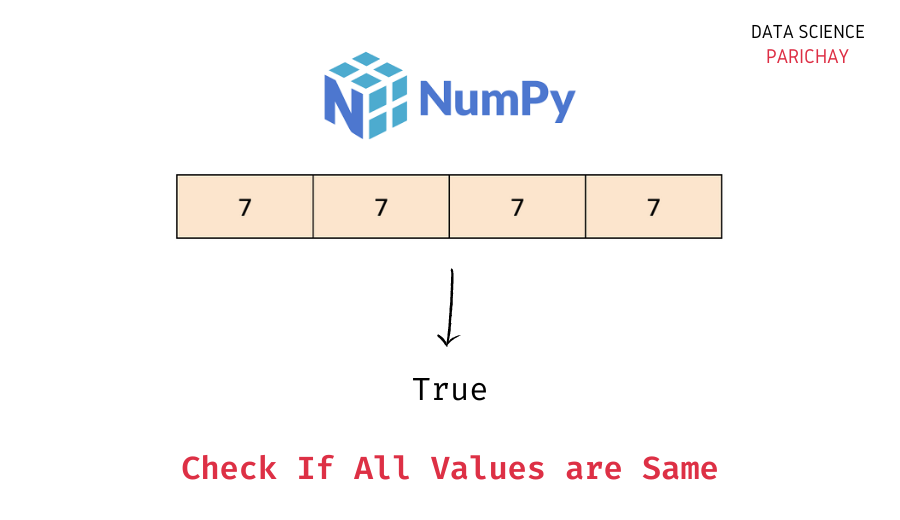# Numpy – Check If All Array Elements are Equal

In this tutorial, we will look at how to check if all the elements in a numpy array are equal or not with the help of some examples.

There are a number of ways to check if a numpy array contains the same value or not –

1. Find the number of unique values in the array and if this number is equal to 1 we can say that all the values in the array are equal.
2. Compare each value in the array to the first value in the array and see if they are all equal or not.

Let’s now look at the two methods with the help of some examples.

## Method 1 – Find the unique values count in the array

If the number of unique values in the array is one, we can say that all the elements in the array are equal.

You can use a combination of the Python `len()` function and the `numpy.unique()` function to get the number of unique values in a numpy array. The following is the syntax –

```# check if all elements are equal in array
len(numpy.unique(ar)) == 1```

Let’s look at an example.

```import numpy as np

# create sample numpy arrays
ar1 = np.array([5, 5, 5])
ar2 = np.array([1, 2, 2])
ar3 = np.array([None])

# check if all elements are equal in array
print(len(np.unique(ar1))==1)
print(len(np.unique(ar2))==1)
print(len(np.unique(ar3))==1)```

Output:

```True
False
True```

We get `True` for the arrays containing only 1 unique value and `False` otherwise.

📚 Data Science Programs By Skill Level

Introductory

Intermediate ⭐⭐⭐

🔎 Find Data Science Programs 👨‍💻 111,889 already enrolled

Disclaimer: Data Science Parichay is reader supported. When you purchase a course through a link on this site, we may earn a small commission at no additional cost to you. Earned commissions help support this website and its team of writers.

Alternatively, you can also create a set from the numpy array and check if the size of the set is 1 or not.

```import numpy as np

# create sample numpy arrays
ar1 = np.array([5, 5, 5])
ar2 = np.array([1, 2, 2])
ar3 = np.array([None])

# check if all elements are equal in array
print(len(set(ar1))==1)
print(len(set(ar2))==1)
print(len(set(ar3))==1)```

Output:

```True
False
True```

We get the same results as above.

## Method 2 – Check if each value in the array is equal to the first value

Here, we compare each value in the array with the first value and check if they are the same or not. If all the values are the same (use the `all()` function), then we can say that all array elements are equal.

Let’s look at an example.

```import numpy as np

# create sample numpy arrays
ar1 = np.array([5, 5, 5])
ar2 = np.array([1, 2, 2])
ar3 = np.array([None])

# function to check all array values are same
def all_array_vals_same(ar):
for i in range(len(ar)):
if ar[i] == ar:
continue
else:
return False
return True

# check if all elements are equal in array
print(all_array_vals_same(ar1))
print(all_array_vals_same(ar2))
print(all_array_vals_same(ar3))```

Output:

```True
False
True```

We get the correct result.

You might also be interested in –

•